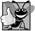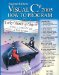# Another C# Application: Adding Integers

### Another C# Application Adding Integers

Our next application reads (or inputs) two integers (whole numbers, like 22, 7, 0 and 1024) typed by a user at the keyboard, computes the sum of the values and displays the result. This application must keep track of the numbers supplied by the user for the calculation later in the application. Applications remember numbers and other data in the computer's memory and access that data through application elements called variables. The application of Fig. 3.18 demonstrates these concepts. In the sample output, we highlight data the user enters at the keyboard in bold.

Figure 3.18. Displaying the sum of two numbers input from the keyboard.

(This item is displayed on page 95 in the print version)

``` 1 // Fig. 3.18: Addition.cs
2 // Displaying the sum of two numbers input from the keyboard.
3 using System;
4
6 {
7 // Main method begins execution of C# application
8 public static void Main( string[] args )
9 {
10 int number1; // declare first number to add
11 int number2; // declare second number to add
12 int sum; // declare sum of number1 and number2
13
14 Console.Write( "Enter first integer: " ); // prompt user
15 // read first number from user
16 number1 = Convert.ToInt32( Console.ReadLine() );
17
18 Console.Write( "Enter second integer: " ); // prompt user
19 // read second number from user
20 number2 = Convert.ToInt32( Console.ReadLine() );
21
22 sum = number1 + number2; // add numbers
23
24 Console.WriteLine( "Sum is {0}", sum ); // display sum
25 } // end method Main
26 } // end class Addition
```

 ```Enter first integer: 45 Enter second integer: 72 Sum is 117 ```

Lines 12

```// Fig. 3.18: Addition.cs
// Displaying the sum of two numbers input from the keyboard.
```

state the figure number, file name and purpose of the application.

Line 5

```public class Addition
```

begins the declaration of class Addition. Remember that the body of each class declaration starts with an opening left brace (line 6), and ends with a closing right brace (line 26).

The application begins execution with method Main (lines 825). The left brace (line 9) marks the beginning of Main's body, and the corresponding right brace (line 25) marks the end of Main's body. Note that method Main is indented one level within the body of class Addition and that the code in the body of Main is indented another level for readability.

Line 10

```int number1; // declare first number to add
```

is a variable declaration statement (also called a declaration) that specifies the name and type of a variable (number1) that is used in this application. A variable is a location in the computer's memory where a value can be stored for use later in an application. All variables must be declared with a name and a type before they can be used. A variable's name enables the application to access the value of the variable in memorythe name can be any valid identifier. (See Section 3.2 for identifier naming requirements.) A variable's type specifies what kind of information is stored at that location in memory. Like other statements, declaration statements end with a semicolon (;).

The declaration in line 10 specifies that the variable named number1 is of type intit will hold integer values (whole numbers such as 7, 11, 0 and 31914). The range of values for an int is 2,147,483,648 (int.MinValue) to +2,147,483,647 (int.MaxValue). We will soon discuss types float, double and decimal, for specifying real numbers, and type char, for specifying characters. Real numbers contain decimal points, as in 3.4, 0.0 and 11.19. Variables of type float and double store approximations of real numbers in memory. Variables of type decimal store real numbers precisely (to 2829 significant digits), so decimal variables are often used with monetary calculations. Variables of type char represent individual characters, such as an uppercase letter (e.g., A), a digit (e.g., 7), a special character (e.g., * or %) or an escape sequence (e.g., the newline character, ). Types such as int, float, double, decimal and char are often called simple types. Simple-type names are keywords and must appear in all lowercase letters. Appendix L summarizes the characteristics of the thirteen simple types (bool, byte, sbyte, char, short, ushort, int, uint, long, ulong, float, double and decimal).

The variable declaration statements at lines 1112

```int number2; // declare second number to add
int sum; // declare sum of number1 and number2
```

similarly declare variables number2 and sum to be of type int.

Variable declaration statements can be split over several lines, with the variable names separated by commas (i.e., a comma-separated list of variable names). Several variables of the same type may be declared in one declaration or in multiple declarations. For example, lines 1012 can also be written as follows:

```int number1, // declare first number to add
number2, // declare second number to add
sum; // declare sum of number1 and number2
```## Good Programming Practice 3 8

Declare each variable on a separate line. This format allows a comment to be easily inserted next to each declaration.## Good Programming Practice 3 9

Choosing meaningful variable names helps code to be self-documenting (i.e., one can understand the code simply by reading it rather than by reading documentation manuals or viewing an excessive number of comments).## Good Programming Practice 3 10

By convention, variable-name identifiers begin with a lowercase letter, and every word in the name after the first word begins with a capital letter. This naming convention is known as camel casing.

Line 14

```Console.Write( "Enter first integer: " ); // prompt user
```

uses Console.Write to display the message "Enter first integer: ". This message is called a prompt because it directs the user to take a specific action.

Line 16

```number1 = Convert.ToInt32( Console.ReadLine() );
```

works in two steps. First, it calls the Console's ReadLine method. This method waits for the user to type a string of characters at the keyboard and press the Enter key to submit the string to the application. Then, the string is used as an argument to the Convert class's ToInt32 method, which converts this sequence of characters into data of an type int. As we mentioned earlier in this chapter, some methods perform a task then return the result of that task. In this case, method ToInt32 returns the int representation of the user's input.

Technically, the user can type anything as the input value. ReadLine will accept it and pass it off to the ToInt32 method. This method assumes that the string contains a valid integer value. In this application, if the user types a noninteger value, a runtime logic error will occur and the application will terminate. Chapter 12, Exception Handling, discusses how to make your applications more robust by enabling them to handle such errors and continue executing. This is also known as making your application fault tolerant.

In line 16, the result of the call to method ToInt32 (an int value) is placed in variable number1 by using the assignment operator, =. The statement is read as "number1 gets the value returned by Convert.ToInt32." Operator = is called a binary operator because it has two operandsnumber1 and the result of the method call Convert.ToInt32. This statement is called an assignment statement because it assigns a value to a variable. Everything to the right of the assignment operator, =, is always evaluated before the assignment is performed.## Good Programming Practice 3 11

Place spaces on either side of a binary operator to make it stand out and make the code more readable.

Line 18

```Console.Write( "Enter second integer: " ); // prompt user
```

prompts the user to enter the second integer. Line 20

```number2 = Convert.ToInt32( Console.ReadLine() );
```

reads a second integer and assigns it to the variable number2.

Line 22

```sum = number1 + number2; // add numbers
```

is an assignment statement that calculates the sum of the variables number1 and number2 and assigns the result to variable sum by using the assignment operator, =. The statement is read as "sum gets the value of number1 + number2." Most calculations are performed in assignment statements. When the application encounters the addition operator, it uses the values stored in the variables number1 and number2 to perform the calculation. In the preceding statement, the addition operator is a binary operatorits two operands are number1 and number2. Portions of statements that contain calculations are called expressions. In fact, an expression is any portion of a statement that has a value associated with it. For example, the value of the expression number1 + number2 is the sum of the numbers. Similarly, the value of the expression Console.ReadLine() is the string of characters typed by the user.

After the calculation has been performed, line 24

```Console.WriteLine( "Sum is {0}", sum ); // display sum
```

uses method Console.WriteLine to display the sum. The format item {0} is a placeholder for the first argument after the format string. Other than the {0} format item, the remaining characters in the format string are all fixed text. So method WriteLine displays "Sum is ", followed by the value of sum (in the position of the {0} format item) and a newline.

Calculations can also be performed inside output statements. We could have combined the statements in lines 22 and 24 into the statement

```Console.WriteLine( "Sum is {0}", ( number1 + number2 ) );
```

The parentheses around the expression number1 + number2 are not requiredthey are included to emphasize that the value of the expression number1 + number2 is output in the position of the {0} format item.Visual C# 2005 How to Program (2nd Edition)
ISBN: 0131525239
EAN: 2147483647
Year: 2004
Pages: 600